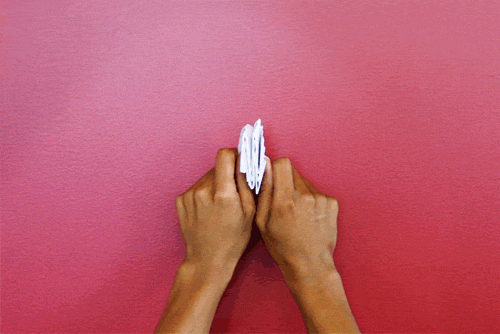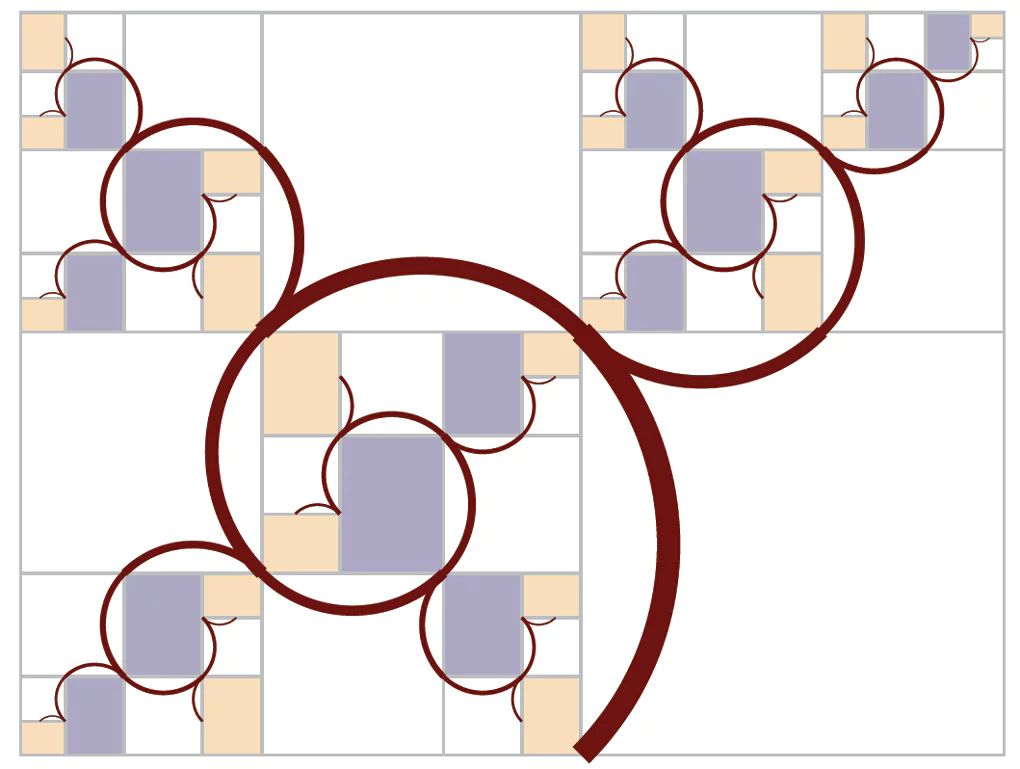# Problems of the Week

Contribute a problem

$\large abc = 10 (a + b + c )$

Let $a,b,c$ be pairwise coprime positive integers greater than 1 that satisfy the equation above. What is the minimum value of $ab+bc+ca$?

A building has a lobby and 10 other floors. 7 people get into the elevator on the lobby, and are uniformly and independently likely to go to any of the 10 floors. They each push the button corresponding to their floor.

To 3 decimal places, what is the expected value of the number of distinct buttons that are pushed?

Find the maximum value of $\ \frac { a }{ c } + \frac { b }{ d } + \frac { c }{ a } + \frac { d }{ b },$ where $a,b,c,d$ vary over all distinct real numbers such that $\frac { a }{ b } + \frac { b }{ c } + \frac { c }{ d } + \frac { d }{ a } = 4$ and $ac = bd$.Consider the animation above, where the rectangular paper is folded and then unfolded repeatedly. They are both done by controlling the opposite vertices of the rectangular paper in a single move.

Assume that the repeating polygons have the same areas and are symmetric. Also assume that horizontal parallel lines are perpendicular to the paper's edges. Which of the following best illustrate the interior creased polygons (including those bounded by the paper's edges) in the animation after unfolding the paper?

Clarification:

• Mix of quadrilaterals refer to class of 4-sided polygons not specified in the options.
• A trapezoid is a quadrilateral with a pair of parallel sides. It is also known as a trapezium in UK.

The Harriss spiral as shown in this graphic is a fractal, where all the white boxes are perfect squares, and all colored boxes are all similar, i.e., even the grey and beige boxes are similar. The entire rectangular enclosure is similar to any of the colored nonwhite boxes.Each colored box only indicates further subdivision by white squares in the same fractal pattern. When reiteration is done infinitely many times, there are only white squares that make up the entire rectangular enclosure.

In each white square there are two brown branches, both quarter circle arcs, but of two different sizes. A botanist decides to study the Harriss [spiral] tree by first cutting all the brown quarter circle segments (BQCS) at the corners of the squares, so that each white square yields 2 BQCSs. He has a row of many boxes. Inside the first box, he puts the one largest BQCS. Inside the second box, he puts in the next largest BQCS. In each box, he puts in all BQCSs of the same size, so that all of the sizes are sorted in a row in progressively smaller sizes, starting with the largest size. The botanist wants to study the distribution of BQCS sizes.

How many BQCSs are there in all of the first 12 sizes, that is, in the first 12 boxes, total?

Note: This Harriss spiral involves the plastic number which is often called the forgotten cousin of the golden ratio.

×